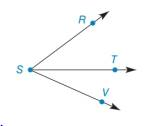Chapter 1.4, Problem 24E### Elementary Geometry for College St...

6th Edition
Daniel C. Alexander + 1 other
ISBN: 9781285195698

#### Solutions

Chapter
Section### Elementary Geometry for College St...

6th Edition
Daniel C. Alexander + 1 other
ISBN: 9781285195698
Textbook Problem
1 views

#Exercises 16 − 24 Given: S T →   bisects  ∠ R S V m ∠ R S T = 2 x + 3 y m ∠ T S V = 3 x − y + 2 m ∠ R S V = 80 ° Find: x     and  y

To determine

To find:

The value of x and y for given information.

Explanation

Given:

STbisects RSVmRST=2x+3ymTSV=3xy+2mRSV=80°

The given figure is,

Definition:

If a point say M lies in the interior of PQR, then mPQM+mRQM=mPQR.

Calculation:

The given figure is,

Figure (1)

Consider the figure (1),

T is the interior point of the angle RSV.

mRST=2x+3ymTSV=3xy+2mRSV=80°

Use above mentioned angle addition postulate to solve for the value of x.

mRST+mTSV=mRSV2x+3y+3xy+2=80

Simplify above equation,

5x+2y=78(1)

It is given that ST is the bisector of RSV

### Still sussing out bartleby?

Check out a sample textbook solution.

See a sample solution

#### The Solution to Your Study Problems

Bartleby provides explanations to thousands of textbook problems written by our experts, many with advanced degrees!

Get Started

#### Find more solutions based on key concepts